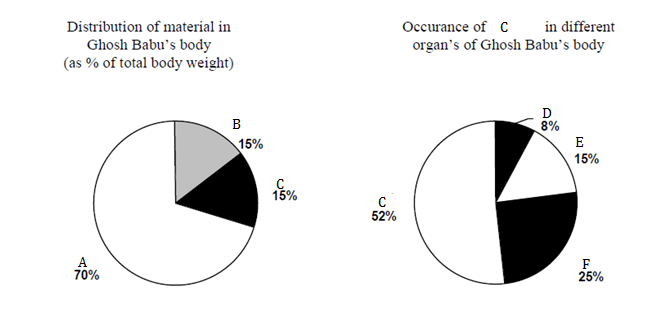Direction for Questions 1 to 4
Refer to the pie-chart given below:Question 1.  What fraction of Ghosh babu’s weight consists of F and D?
(a) 1/13
(b) 1/30
(c) 1/20
(d) Cannot be determined

Question 2.  Ratio of distribution of Cin F to the distribution of C in D is
(a) 25: 3
(b) 3 : 10
(c) 1 : 3
(d) 31/2 : 1

Question 3.  How much of Ghosh Babu’s body weight is made up of D?
(a) 0.15
(b) 10
(c) 1.2
(d) Cannot be determined

Question 4. In terms of total body weight, the portion of material other than A and C is closest to
(a) 3/20
(b) 1/15
(c) 85/100
(d) 1/20

From the pie chart we can say thatthe percentage of the total protein constituted by the D & F is 33 %

Percentage of C in the body = 15 % of the total body weight

Therefore percentage of D & F as a fraction of the total body weight = 33% of 15% = 5 %. = 1/20.

Ratio of distribution of C in F to the distribution of C in D is

= 25 : 8.